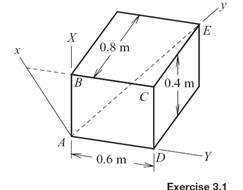### Create an Account

Home / Questions / Solve Exercise 31 by considering the transformation from XYZ to xyz to be the result of a ...

# Solve Exercise 31 by considering the transformation from XYZ to xyz to be the result of a sequence of bodyfixed rotations Exercise 31 The XYZ coordinate system coincides with the edges of the

Solve Exercise 3.1 by considering the transformation from XYZ to xyz to be the result of a sequence of body-fixed rotations.

Exercise 3.1

The XYZ coordinate system coincides with the edges of the box, the y axis coincides with the main diagonal for the box, and the x axis coincides with face ABCD. Use the orthonormal properties to determine the transformation that converts vector components relative to XYZ to components relative to xyz. Then use this transformation to determine the xyz coordinates of corner C.Jun 18 2020 View more View LessSubscribe To Get Solution